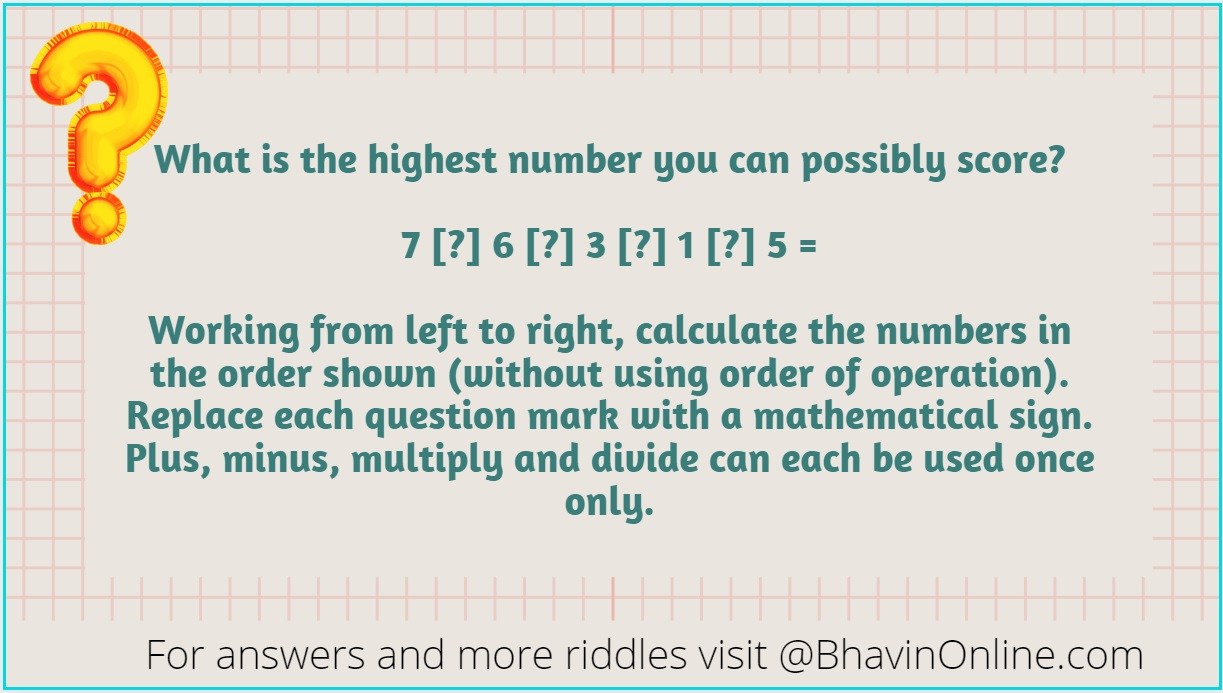# What Is the Highest Number You Can Possibly Score: 7 [?] 6 [?] 3 [?] 1 [?] 5 = ?

Have fun with numbers with this riddle.

What is the highest number you can possibly score?

7 [?] 6 [?] 3 [?] 1 [?] 5 = [?]

Working from left to right, calculate the numbers in the order shown (without using order of operation).

Replace each question mark with a mathematical sign.

Plus, minus, multiply and divide can each be used once only.So were you able to solve the riddle? Leave your answers in the comment section below.